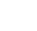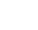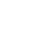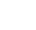# How are point objects distributed in space? Finding an answer with spatial statistics, Clark-Evans testThis post begins a series of posts on the spatial statistics techniques. Spatial statistics is a cross-disciplinary field between geoinformatics and statistics. Its methods are useful at spatial data analysis that is analyzing the data of spatially coordinated observations. If a geographical location of observations matters, this makes a very case for the application of spatial statistics techniques. They will aid finding patterns in objects and traits distribution, describing object interrelations and reveal the structure of spatial data.

At the beginning, we will illustrate the use of spatial statistics techniques following the examples of ArcGIS applications. Further on we are going to post more on this topic concerning the use of QGIS and R applications.

### Theory

Vector layers with point geometry are the data that all GIS users deal with. In the Environmental and Earth Sciences, a lot of such data were processed at early stages of the Global Positioning Systems (GPS) usage (GNSS-receivers). These could be coordinates of sampling points, measurement sites, locations of some animal and plant species observations, etc. In Social, Economic Sciences and Humanities GIS are applied as well to analyze point source data that could be, for example, criminal incident, traffic collision, and accident sites.

The first question that arises while analyzing point data is how these objects are spatially distributed. Cartographic visualization is not able to provide an unbiased answer since visual analysis of maps is rather a subjective procedure. Image perception differs between different observers so conclusions may differ, too. One should get a quantitative evaluation technique. Such a technique is represented with the Clark-Evans criterion (i.e. the nearest neighbor index).

This index was developed by two scientists, Philip Clark and Francis Evans who worked at the Institute of Human Biology, The University of Michigan. First, this technique was described in their article published in 1954 in the American journal ‘Ecology’.

There is a countless set of cases how objects can be distributed in the geographic space. However, these could be grouped into three major types: even (dispersed), random (chaotic), and aggregated (clustered) distribution (fig. 1). The Clark-Evans criterion helps to identify such a type of spatial distribution of the studied objects.##### Fig. 1. Types of spatial distribution of objects (from left to right): even, random and aggregated

Let’s get started with the calculation of the Clark-Evans criterion. This is quite easy. You have to make several operations. Formulas for these operations use the following notations:

n – total number of objects,

r – the nearest neighbor distance for a certain object,

S – total surveyed area,

ρ – object density,– actual (measured) mean nearest neighbor distance,– expected (theoretically) mean nearest neighbor distance,

R – the Clark-Evans criterion,

z – z-criterion used for statistical significance proof,– the standard error of calculated expected mean nearest neighbor distance.

1) First, for each object, the nearest neighbor should be identified and a nearest neighbor distance r is measured.

2) The actual mean nearest neighbor distance () is calculated as a sum of all measured nearest neighbor distances divided by the total number of objects:3) The expected mean nearest neighbor distance at random distribution of objects is calculated as:4) Then the ratio of the actual to the expected nearest neighbor distance is calculated that is the Clark-Evans criterion (R):After having the criterion calculated it should be interpreted. If the Clark-Evans criterion equals 1 then spatial distribution type is random (chaotic), i.e. null-hypothesis for the criterion. If it is greater than 1, the distribution is even (dispersed). And if the Clark-Evans criterion is less than 1, the distribution is aggregated (clustered).

However, here is a nuance. How big should be the difference between the calculated R and 1 to tell the Clark-Evans criterion differs from 1? To assess the statistical significance of such a difference the z-criterion should be calculated:After calculating z-criterion it should be compared to its standard value (given in the relevant statistical tables) at certain significance level. But practically few ones do it in such a way. Modern software is giving us an exact probability of the first-type error (p-value). If it is less than 0,05 it means we have a statistically significant difference between the calculated R and 1.

In conclusion, one should say that the Clark-Evans criterion can be calculated not only for point objects but for polygonal and multilinear objects, as well. In these cases calculations are done using the objects’ centroid coordinates.

### Practice

Now we get started with the application of the Clark-Evans criterion in the ArcGIS environment.

We will use the following case. Our data are settlements in the five administrative districts of the Belgorod region, namely the Belgorod, Borisovka, Korocha, Shebekine, and Yakovlivka Districts (fig. 2). This is a territory occupied by the Belgorod Metropolitan Area. We have to identify the type of settlements spatial distribution.##### Fig. 2. Settlement locations in the five districts of Belgorod region (from top to down, left to right: Yakovlivka, Korocha, Borisovka, Belgorod, and Shebekine Districts)

1) Select from the Toolbox (ArcToolbox) the command Spatial Statistic ToolsAnalyzing PatternsAverage Nearest Neighbor (fig. 3).##### Fig. 3. Selecting the command in the ArcToolbox

2) There appears the window Average Nearest Neighbor (fig. 4), in which you should adjust the procedure parameters:##### Fig. 4. The window for adjusting parameters to calculate the Clark-Evans criterion

3) Identify the vector layer containing the data to be analyzed in the field Input Feature Class;

4) Select the distance measuring method in the field Distance Method. There are two methods – the Euclidean and the Manhattan (City Block) Distance (fig. 5).

Euclidean distance is a common distance measured as the straight line connecting two objects. In our case, one should select namely the Euclidean distance.

Manhattan distance is a sum of catheti of the orthogonal triangle the hypotenuse of which is a straight line connecting two objects. In other words, this is a sum of absolute differences between the coordinates on two axes. We deal with such a distance in everyday life, for example, while walking by the urban streets. In this case, we often cannot walk straightforward because we could rest ourselves on a wall. We need to pass round the building, first to go to a crossing and then to turn in a right direction. That is why the other name of this distance is the City Block distance. The name Manhattan distance, in turn, was given from the famous part of New York City, Manhattan, that is featuring orthogonal street plan.##### Fig. 5. Euclidean (left) and Manhattan (right) distances

5) Set the area in the field Area. It should be measured in the same units as the data frame. In our case, it is a square meter. The area of the five districts equals 6 698 031 060,357 m2.

The Area is not a mandatory field to fill-in. It is set to empty by default, and the area is taken as equal to the area of a square drawn through the most distant points. If the shape of the studied territory is approximated by a rectangle the default settings can be used. In other cases, the exact area should be set manually in order to avoid calculation errors.

On the fig. 6 (right picture) the example is given to show that default area settings may lead to erroneous results. Instead of the even mode of distribution, we could detect the random one. In contrast, the picture on the left shows an example when both variants give the same result – the even distribution.##### Fig. 6. The cases when using the default area settings does not lead to errors (picture on the left) and when it leads to errors (picture on the right)

Legend: circular dots – point objects; solid line outlines the minimal rectangle approximating the studied territory; dotted line outlines actual boundaries of the studied territory.
6) Specify whether or not to generate a graphical report (tumbler Generate Report). The results of calculations are shown by default in the message that appears upon procedure completion. But the program can generate also a graphical report as an html-file. It contains not only the calculation results but their interpretation. Thus fig. 7 shows a graphical report for our case. The frame outlines a correct type of spatial distribution for the data processed. This is an even (dispersed) distribution. And this is the result that was expected. Indeed, the Belgorod Metropolitan Area is a territory where settlements are distributed evenly.##### Fig. 7. The message and graphical report on the calculation results for the nearest neighbor analysis

One should take in mind that at producing a graphic report the ArcGIS program rejects null-hypothesis at p<0,05. If you need a stronger statistical confidence (for example to reject null-hypothesis at p<0,01 or p<0,001), you have to interpret the results by yourself according to numerical results obtained.

Let us proceed with the numerical results obtained for our case. We see that observed mean nearest neighbor distance is 2198,41 m. By the way, what does it mean for a layperson? Given an average pedestrian’s speed at 6 km/h, in our case, he/she could get to one settlement point from another in about 22 minutes. Of course, if a direct road is available, the weather conditions are favorable and there are no serious obstacles to the movement.

At random distribution mode of objects on such an area, an expected mean nearest neighbor distance should equal 2013,58 m. This is less than observed mean distance. So, when we divide actual mean distance to expected one we get the Clark-Evans criterion equal to 1,09.

The criterion is greater than 1. But is this difference sufficient to tell the even mode of settlement distribution from the random mode? The z-test gives p = 0,0004. This means a very low probability of mistake when rejecting null-hypothesis (that is a random distribution). Then, we reject the null hypothesis and accept the alternative that settlements are distributed evenly.